# NEET Questions Solved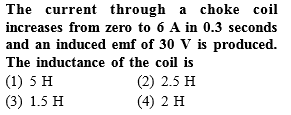Complete Question Bank + Test Series
Complete Question Bank

Difficulty Level: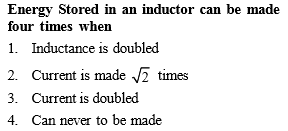Complete Question Bank + Test Series
Complete Question Bank

Difficulty Level: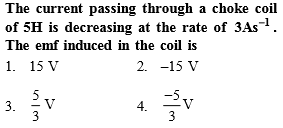Complete Question Bank + Test Series
Complete Question Bank

Difficulty Level:

If all linear dimensions of an inductor are tripled, then self inductance will become (keeping total number of turns per unit length constant)

1.  3 times

2.  9 times

3.  27 times

4. $\frac{1}{3}$ times

Complete Question Bank + Test Series
Complete Question Bank

Difficulty Level: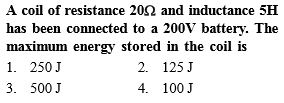Complete Question Bank + Test Series
Complete Question Bank

Difficulty Level:

The self inductance of a coil is L. Keeping the length and area same, the number of turns in the coil is increased to four times. The self inductance of the coil will now be

(1) $\frac{1}{4}L$

(2) L

(3) 4 L

(4) 16 L

Complete Question Bank + Test Series
Complete Question Bank

Difficulty Level:

Pure inductance of 3.0 H is connected as shown below. The equivalent inductance of the circuit is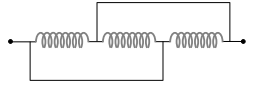(1) 1 H

(2) 2 H

(3) 3 H

(4) 9 H

Complete Question Bank + Test Series
Complete Question Bank

Difficulty Level:

If a current of 10 A flows in one second through a coil, and the induced e.m.f. is 10 V, then the self-inductance of the coil is

(1) $\frac{2}{5}H$

(2) $\frac{4}{5}H$

(3) $\frac{5}{4}H$

(4) 1 H

Complete Question Bank + Test Series
Complete Question Bank

Difficulty Level:

The adjoining figure shows two bulbs B1 and B2 resistor R and an inductor L. When the switch S is turned off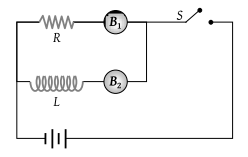(1) Both B1 and B2 die out promptly

(2) Both B1 and B2 die out with some delay

(3) B1 dies out promptly but B2 with some delay

(4) B2 dies out promptly but B1 with some delay

Complete Question Bank + Test Series
Complete Question Bank

Difficulty Level:

In the figure magnetic energy stored in the coil is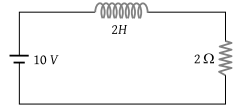(1) Zero

(2) Infinite

(3) 25 joules

(4) None of the above